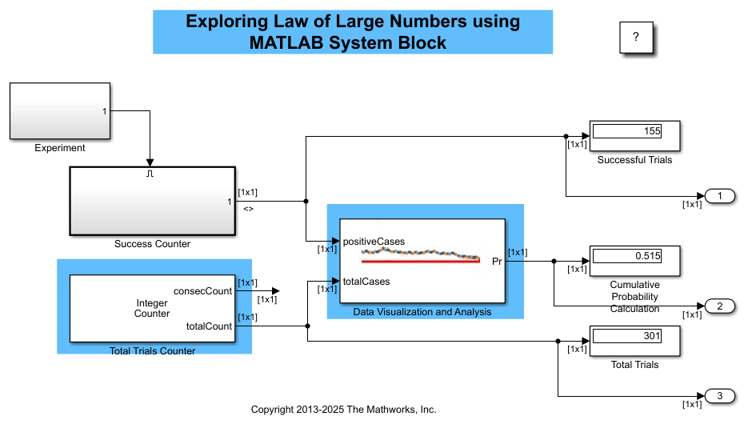# Illustration of Law of Large Numbers

This example shows how to use MATLAB System blocks to illustrate the law of large numbers.

The law of large numbers states that the cumulative average probability of an event approaches the expected value as the number of trials approaches infinity. You can see this in the generated plot.

A Uniform Random Number block connected to Compare To Constant block acts as a Bernoulli trial. The example uses the high value as successful outcome of the experiment.

This example highlights the use of MATLAB System block as follows:

• The Success Counter counts the number of successful trials. The Total Trials Counter counts the total number of trials. Both blocks use the same System object™. This System object is a source block that uses the "resetImpl" method to count the number of times the block has run at consecutive time steps.

• The Analysis and Plot block calculates the average probability and plots it. It uses a System object that inherits from the interface classes matlab.System. The interface class matlab.System is required for all System objects. To use features not supported for code generation, such as unsupported functions, define propagator methods for the System object. As a result, this block cannot simulate in "Code generation" mode. It must simulate in "Interpreted execution" mode.

• The two MATLAB System blocks use custom icons. The Analysis and Plot block uses an image file icon. The Total Trials Counter block uses a multiline text icon. The "getIconImpl" methods of the corresponding System objects define these custom icons.